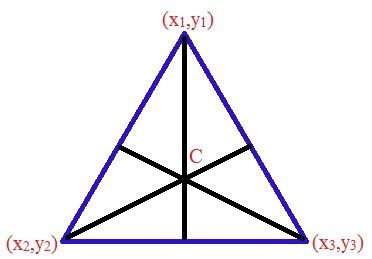# Centroid Formula

## What is Centroid

Centroid is the geometrical concept which refers to its geometric center of the object.

Centroid formula is used to determine the coordinates of a triangle’s centroid. The centroid of a triangle is the center of the triangle, which can be determined as the point of intersection of all the three medians of a triangle. The median is a line drawn from the midpoint of any one side to the opposite vertex. It should be noted that the centroid divides all the medians of the triangle in 2:1 ratio.Centroid of the Triangle

### Centroid Formula

The Centroid Formula is given by

 Formula for Centroid C = [(x1 + x2 + x3)/ 3, (y1 + y2 + y3)/ 3]

Where,

• C denotes centroid of the triangle.
• x1, x2, x3 are the x-coordinates of the vertices of a triangle.
• y1, y2, y3 are the y-coordinates of the vertices of a triangle.

### Solved Examples

Example 1:

Determine the centroid of a triangle whose vertices are (5,3), (6,1) and (7,8).

Solution

Given parameters are,

(x1, y1) = (5,3)

(x2, y2) = (6,1)

(x3, y3) = (7,8)

The centroid formula is given by

C = [(x1 + x2 + x3)/ 3, (y1 + y2 + y3)/ 3)

C = [(5 + 6 + 7) / 3, (3 + 1 + 8) / 3]

C = (18 / 3, 12 / 3)

C = (6, 4)

Example 2:

Calculate the centroid of a triangle whose vertices are (9,0), (2,8) and (1,4).

Solution

Given parameters are

(x1, y1) = (9, 0)

(x2, y2) = (2, 8)

(x3, y3) = (1, 4)

The centroid formula is given by,

C = [(x1 + x2 + x3)/ 3, (y1 + y2 + y3)/ 3]

C = [(9 + 2 + 1) / 3, (0 + 8 + 4) / 3]

C = (12 / 3, 12 / 3)

C = (4, 4)

Example 3: If the (2, 2) are coordinates of the centroid of a triangle whose vertices are (0, 4), (-2, 0) and (p, 2), then find the value of p.

Solution:
Given vertices of a triangle are:
(x1, y1) = (0, 4)
(x2, y2) = (-2, 0)
(x3, y3) = (p, 2)
Centroid = (x, y) = (2, 2)

Using the centroid formula,

(x, y) = [(x1 + x2 + x3)/3, (y+ y2 + y3)/3]

(2, 2) = [(0 – 2 + p)/3, (4 + 0 + 2)/3]

(2, 2) = [(p-2)/3, 6/3)]

Equating the x-coordinates,
(p – 2)/3 = 2
p – 2 = 2 × 3
p = 6 + 2
p = 8
Hence, the value of p = 8

Stay tuned with BYJU’S for more such interesting articles.